Courses

# Bearings - Mechanical Engineering - 3

## 30 Questions MCQ Test Machine Design | Bearings - Mechanical Engineering - 3

Description
This mock test of Bearings - Mechanical Engineering - 3 for Mechanical Engineering helps you for every Mechanical Engineering entrance exam. This contains 30 Multiple Choice Questions for Mechanical Engineering Bearings - Mechanical Engineering - 3 (mcq) to study with solutions a complete question bank. The solved questions answers in this Bearings - Mechanical Engineering - 3 quiz give you a good mix of easy questions and tough questions. Mechanical Engineering students definitely take this Bearings - Mechanical Engineering - 3 exercise for a better result in the exam. You can find other Bearings - Mechanical Engineering - 3 extra questions, long questions & short questions for Mechanical Engineering on EduRev as well by searching above.
QUESTION: 1

### Tapered roller bearing can take

Solution:

Tapered roller can take both redial and axial load.
But Fr > Ra
and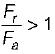where Fr = radial load and Fa = Axial load

QUESTION: 2

### Which of the following are antifriction bearing

Solution:

Since coefficient of friction (μ) for Ball and Roller bearings are very low as compared to sliding contact bearing hence they are known as antifriction bearing.

QUESTION: 3

### Bearing used for heavy loads are

Solution:

Roller bearing are suitable for heavy loads ball bearing may deform at heavy loads.

QUESTION: 4

In a journal bearing, the radius of the friction circle increases with increase in

Solution:

Radius of friction circle = μ x r
where μ = Coefficient of friction
r = Radius of journal

QUESTION: 5

Which one of the following types of bearings is employed in shafts of gear boxes of automobiles

Solution:
QUESTION: 6

In a single row deep groove ball bearing cage is needed to

Solution:

Cage is used in the bail bearing to prevent the cluster of balls or roiling element.

QUESTION: 7

What is the main advantage of hydrodynamic bearing over roller bearing

Solution: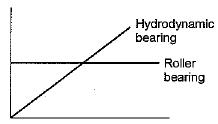As the speed increases, load carrying capacity of hydrodynamic bearing increases.

QUESTION: 8

In hydrodynamic bearings

Solution:

Hydrodynamic bearing is a thick film lubrication bearing in which oil pressure is generated due to rotation of shaft.

QUESTION: 9

In hydrostatic bearings

Solution:

Hydrostatic bearing is also a thick film lubrication bearing in which oil pressure is created by some external means like pump.

QUESTION: 10

Which of the following bearing is preferred for oscillating conditions

Solution:

Needle roller bearings are ideally suited for application involving oscillatory motion such as piston pin bearings, rocker arms and universal joint, They are also suitable for continuous rotation where the load is variable or intermittent.

QUESTION: 11

Cage is not required in

Solution:

Since in needle roller bearing
l/d is very-very large hence cage is not required in needle roller bearing.

QUESTION: 12

Which one of the following is known as maximum capacity bearing?

Solution:
QUESTION: 13

If K = 3 for ball bearing and K = 3.33 for roller bearings. Which one of the following correctly states load (p) life (L) relationship for rolling contact bearings?

Solution:
QUESTION: 14

The bearing characteristic number in a hydrodynamic bearing depends upon

Solution:

Bearing characteristic number = ZN/P
Hence, it is function of viscosity, speed and bearing pressure.

QUESTION: 15

Which one of the following bearing is suitable for the application where large radial load at very high speed is use

Solution:

The load carrying capacity of sliding contact bearing (Hydrodynamic bearing) is linearly proportional to speed while rolling contact bearing have finite life for a given combination of load and speed. Sliding contact bearing is suitable for high load high speed condition particularly from the consideration of a long life.

QUESTION: 16

Which one of the following is criterion in the design of hydrodynamic journal bearings

Solution:

Sommerfeld number is used in the designing of hydrodynamic bearing while dynamic capacity static capacity is the term used in roiling contact bearing.

QUESTION: 17

In sliding contact bearings, a positive pressure can be built up and a load supported by a fluid only by the use of a

Solution: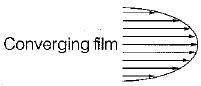QUESTION: 18

It is seen from the curve that there is a minimum value of the coefficient of friction (m) for a particular value of the Bearing Characterstic Number denoted by a. What is this value of the Bearing Characteristic Number called?

Solution:

Bearing modulus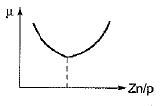QUESTION: 19

A ball-bearing is characterized by basic static capacity = 11000 N and dynamic capacity = 18000 N. This bearing is subjected to equivalent static load = 5500 N. The bearing loading ratio and life in million revolutions respectively are

Solution:

Loading ratio = C/P = 18000/5500 = 3.27
Life (million revolutions)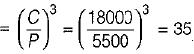QUESTION: 20

Consider the following statements:
For a journal rotating in a bearing under film lubrication conditions, the frictional resistance is
1. Proportional to the area of contact
2. Proportional to the viscosity of lubricant
3. Proportional to the speed of rotation
4. Independent of the pressure
Q. Which of the statements given above are correct?

Solution: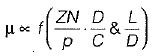Frictional resistance ∝ Z
∝ N
∝ 1/p
So 2 or 3 is correct.

QUESTION: 21

In three ball bearings identified as SKF 2015, 3115 and 4215

Solution:

According to ISO plan for dimension series bearings are provided with two digit numbers, The first number indicate the width series 8, 0, 1, 2, 3, 4, 5 and 6 in order of increasing width. The second number indicate diameter series 7, 8, 9, 0, 1, 2, 3 and 4 in order of ascending outer diameter of bearing. Thus bearing number SKF 2015, 3115 and 4215 shows bearings belonging to different series with 75 mm bore diameter but width is increasing.
SKF 2015, 3115 and 4215 shows width is increasing ascending outer diameter of bearing same bore diameter 75 mm. (i.e. 15 x 5).

QUESTION: 22

A journal has a shaft diameter of 40 mm and a length of 40 mm. The shaft rotating at 20 rad/s and the viscosity of the lubricant is 20 MPa.s. The clearance is 0.20 mm. The loss of torque due to the viscosity of the lubricant is approximately

Solution: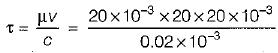= 400 N/m2
Force
= τA
= 400 x 3.14 x 0.04 x 0.04 N
(∵ F = τ·πdl)
Torque = 400 x 3.14 x 0.04 x 0.04 x 0.02
(∵ torque = Fx r) = 0.040 Nm

QUESTION: 23

A natural feed journal bearing of diameter 50 mm and length 50 mm operating at 20 revolution/sec. carries a load of 2.0 kN. The lubricant used has a viscosity of 20 MPa/s. The radial clearance is 50 μm. The Sommerfield number for the bearings is

Solution:

Sommerfield number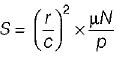Where, r is radius of journal
μ is viscosity of lubricant
N is number of revolution per second
ρ is bearing pressure on projected Area
C is radial clearance
Therefore,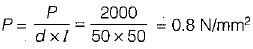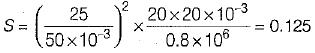QUESTION: 24

The dynamic load capacity of 6306 is 22 kN. The maximum radial load it can sustain to operate at 600 rev/min, for 2000 hours is

Solution:

Life of bearing in millions of revolution
= 200 x 60 x 600
L = 72 millions of Revolution
Radial load capacity = 22 kN
We have for ball bearing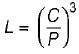C = Dynamic load
P = Static load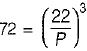P = 5.28 kN

QUESTION: 25

The basic load rating of a ball bearing is

Solution:
QUESTION: 26

The life of a ball bearing at a load of 10 kN is 8000 hours. Its life in hours, if the load is increased to 20 kN, keeping all other conditions the same, is

Solution: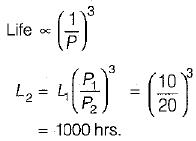QUESTION: 27

Consider the following statements:
For a proper hydrodynamic lubrication for a given journal bearing
1. The higher the viscosity, the lower the rotating speed needed to float the journal at a given load.
2. The higher the rotating speed, the higher the bearing load needed to float the journal at a given viscosity.
3. The higher the bearing load, the higher the viscosity needed to float the journal at a given speed.
Q. Which of these statement are correct?

Solution:
QUESTION: 28

In a hydrodynamic journal bearing, there is

Solution:
QUESTION: 29

Consider the following statements:
The fatigue ‘L’ of rolling bearing is
1. Inversely proportional to the third power of the load for ball bearing.
2. Inversely proportional to the fourth power of the load for ball bearing.
3. Inversely proportional to the 10/3 power for roller bearing.
4. Inversely proportional to the third power of the load for roller bearing.

Solution:

Life of Bearing in millions of revolution for roller bearing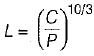Life of bearing in millions of revolution for ball bearing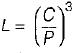QUESTION: 30

Which one of the following statement is not true of rolling contact bearing?

Solution:

1st statement is not valid for rolling contact bearing it is useful in journal bearing.